# The numbers in the blocks given below follow a certain pattern. Study the pattern and choose the number which will replace the question mark?

2019-09-03 | Team PendulumEdu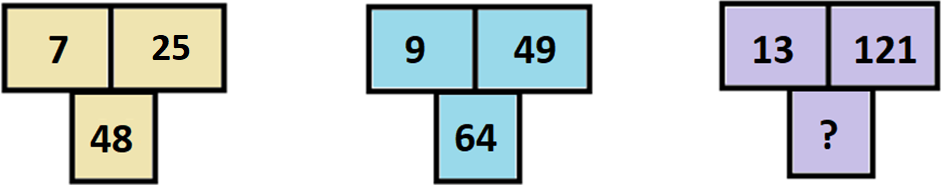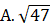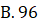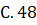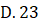Solution:

Observing the number in the first block we get the pattern as,

(7)2 – 25 = 24

And, 2 * 24 = 48

Similarly, observing the number in the second block we get the pattern as,

(9)2 – 49 = 32

And, 2 * 32 = 64

Therefore, considering the third block we get,

(13)2 – 121 = 169 – 121 = 48

So,

? = 2 * 48 = 96

Hence, (B) is the correct answer.

Such type of question is asked in various competitive exams like SSC CGL, SSC MTS, SSC CPO, SSC CHSL, RRB JE, RRB NTPC, RRB GROUP D, etc. Try and attempt free mock tests at PendulumEdu and learn easy techniques to solve such questions. Also, one can clear all exam related doubts through PendulumEdu’s one-to-one session.0